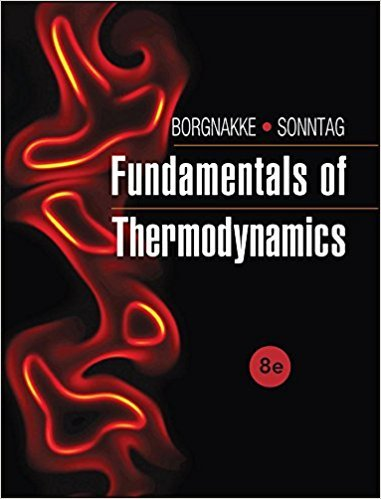×
Get Full Access to Fundamentals Of Thermodynamcs - 8 Edition - Chapter 3 - Problem 23cgp
Get Full Access to Fundamentals Of Thermodynamcs - 8 Edition - Chapter 3 - Problem 23cgp

×

# A rigid tank with pressurized air is used (a) to increaseISBN: 9781118131992 58

## Solution for problem 23CGP Chapter 3

Fundamentals of Thermodynamcs | 8th Edition

• Textbook Solutions
• 2901 Step-by-step solutions solved by professors and subject experts
• Get 24/7 help from StudySoup virtual teaching assistantsFundamentals of Thermodynamcs | 8th Edition

4 5 1 323 Reviews
18
4
Problem 23CGP

Problem 23CGP

A rigid tank with pressurized air is used (a) to increase the volume of a linear spring-loaded piston/ cylinder (cylindrical geometry) arrangement and (b) to blow up a spherical balloon. Assume that in both cases P = A + BV with the same A and B. What is the expression for the work term in each situation?

Step-by-Step Solution:

Solution 23CGP

Step 1 of 4

The objective here is to derive an expression for the work done in the given scenarios, when the relation between pressure and volume is given.

Step 2 of 4

Step 3 of 4

##### ISBN: 9781118131992

Unlock Textbook Solution